# Square root function

To use this function, choose Calc > Calculator.

For any nonnegative number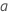, the square root is the nonnegative number n such that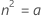. The square root can be represented by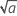or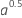.

For example,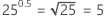.

## Syntax

SQRT(number)

For number, specify the number or column of numbers. If you enter a negative number, Minitab returns a missing value.

## Examples

Calculate expression Result
SQRT(64) 8
SQRT(0.25) 0.5

## Uses

The square root has many applications in statistics. For example:
• To estimate the standard deviation in regression, Minitab calculates the square root of the mean square of the error (MSE).
• To transform skewed data using the Box-Cox transformation (with lambda = 0.5), Minitab calculates the square root of the data values.
• To make a box or range plot width proportional to the sample size, Minitab makes the width of each box proportional to the square root of the number of observations in the box.
By using this site you agree to the use of cookies for analytics and personalized content.  Read our policy